Journal of Integer Sequences, Vol. 1 (1998), Article 98.1.5

## The Number of Triangles Formed by Intersecting Diagonals of a Regular Polygon

### Steven E. Sommars Lucent Technologies Indian Hill, IL Email address: sommars@enteract.com and Tim Sommars Wheaton North High School Wheaton, IL Email address: ozzy50@juno.com

(Affliliation for identification only, the paper is not officially endorsed by either organization.)

Abstract: We consider the number of triangles formed by the intersecting diagonals of a regular polygon. Basic geometry provides a slight overcount, which is corrected by applying a result of Poonen and Rubinstein . The number of triangles is 1, 8, 35, 110, 287, 632, 1302, 2400, 4257, 6956 for polygons with 3 through 12 sides.

Introduction

If we connect all vertices of a regular N-sided polygon we obtain a figure with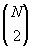= N (N - 1) / 2 lines. For N=8, the figure is: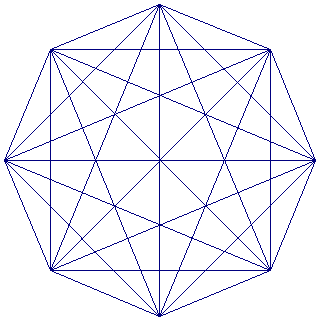Careful counting shows that there are 632 triangles in this eight sided figure.

Derivation

All triangles are formed by the intersection of three diagonals at three different points. There are five arrangements of three diagonals to consider. We classify them based on the number of distinct diagonal endpoints. We will directly count the number of triangles with 3, 4 and 5 endpoints (top three figures). We will count the number of potential triangles with 6 endpoints, then correct for the false triangles. In each of the following five figures, a sample triangle is highlighted.

Three, Four and Five Diagonal Endpoints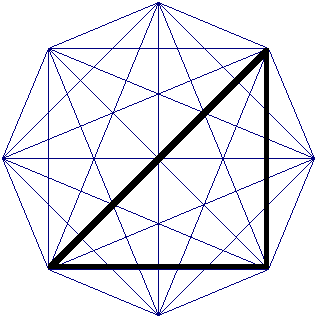3 diagonal endpoints. There are 56 such triangles in the figure at left.   The number of triangles formed by diagonals with a total of three endpoints is simply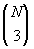.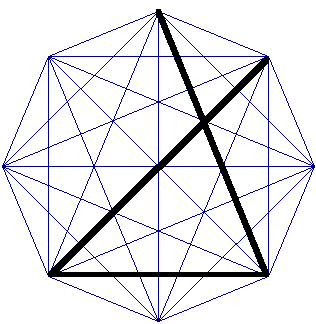4 diagonal endpoints. There are 280 such triangles in the figure at left.   There are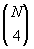combinations of the four diagonal endpoints. For each set of four endpoints, there are four triangle configurations. Thus there are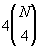triangles formed.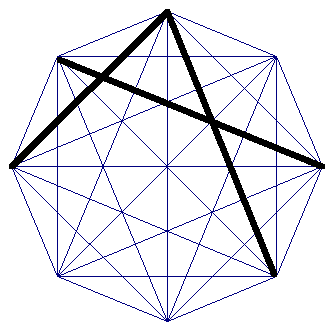5 diagonal endpoints There are 280 such triangles in the figure at left. For each of the N vertices of the polygon, there are four other diagonal endpoints which can be placed on the N-1 remaining locations. Thus there are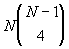triangles formed. This is equal to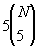.

Six diagonal endpoints

The number of potential triangles formed by 6 line segments is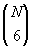, since there are 6 segment endpoints to be chosen from a pool of N. Often potential triangles are not created by three overlapping line segments because the line segments intersect at a single point.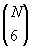counts both of the following two situations.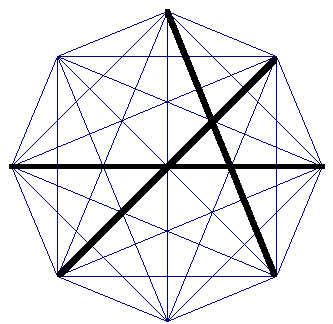6 diagonal endpoints, resulting in triangle. There are 16 such triangles in the figure at left.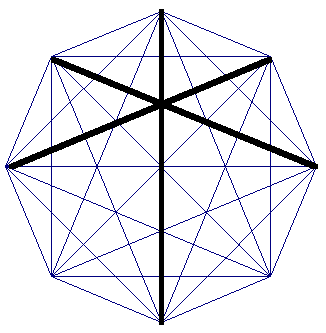6 diagonal endpoints, false triangle. There are 9 interior intersection points in the figure at left where such false triangles can be formed.

We use a result of  to count these false triangles. As in that paper, for a regular N-sided polygon, let am(N) denote the number of interior points other than the center where m diagonals intersect. Surprisingly, only the values m = 2, 3, 4, 5, 6 or 7 may occur. The requisite formulae from  are reproduced here: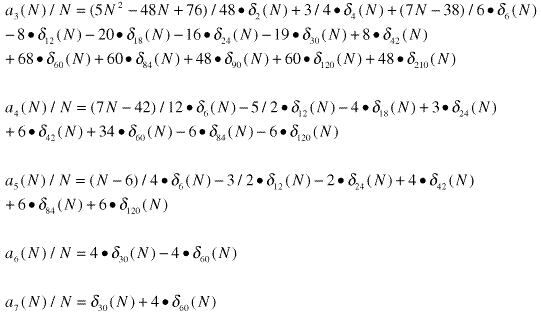where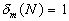if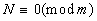, 0 otherwise.

If there are K line segments that intersect at one common point, where K>2, there are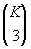false triangles corresponding to that point. Thus the correction term for false triangles is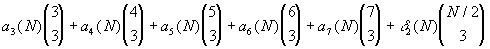where the last term represents the contribution of the center point for even N. The correction is 0 for odd N. The number of triangles formed by line segments with six endpoints on the polygon is then: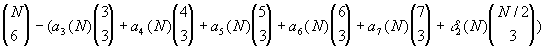Result

The table below summaries the results for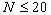. These values were checked through use of a computer program performing an exhaustive search.

 N Triangles with 3 diagonal endpoints Triangles with 4 diagonal endpoints Triangles with 5 diagonal endpoints Triangles with 6 diagonal endpoints Total Number of Triangles 3 1 0 0 0 1 4 4 4 0 0 8 5 10 20 5 0 35 6 20 60 30 0 110 7 35 140 105 7 287 8 56 280 280 16 632 9 84 504 630 84 1302 10 120 840 1260 180 2400 11 165 1320 2310 462 4257 12 220 1980 3960 796 6956 13 286 2860 6435 1716 11297 14 364 4004 10010 2856 17234 15 455 5460 15015 5005 25935 16 560 7280 21840 7744 37424 17 680 9520 30940 12376 53516 18 816 12240 42840 17508 73404 19 969 15504 58140 27132 101745 20 1140 19380 77520 38160 136200

The sequence formed by the total number of triangles was studied by the late Victor Meally in the 1960's, although it appears he did not find our formula for the N-th term. This is sequence A006600 in the On-Line Encyclopedia of Integer Sequences.

To summarize the final result, the number of triangles generated by intersecting diagonals of an N-regular polygon is: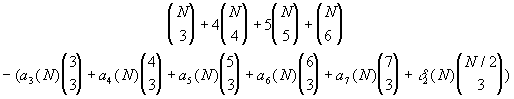References

 Bjorn Poonen, Michael Rubinstein, The Number of Intersection Points Made by the Diagonals of a Regular Polygon, SIAM J. Disc. Math. 11 (1998), 133-156. Note that Theorem 1 has a typographical error: in the second line: 232 should be replaced by 262.

Received February 24 1998; published in Journal of Integer Sequences, April 26 1998.